# Types of Angles- Acute, Obtuse, Right, Straight, Reflex, Complete and Zero Angle.

## Notes

### Types of Angles:

1. An acute angle measures between 0° and 90°.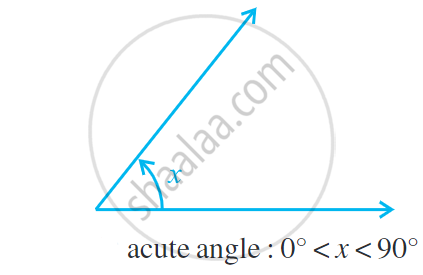2. The right angle is exactly equal to 90°.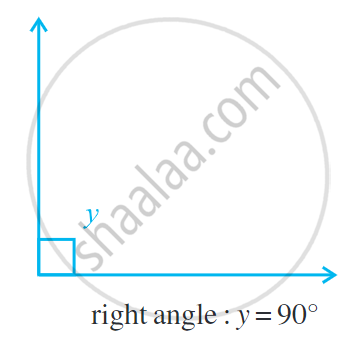3. An angle greater than 90° but less than 180° is called an obtuse angle.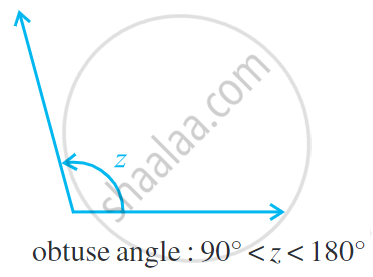4. A straight angle is equal to 180°.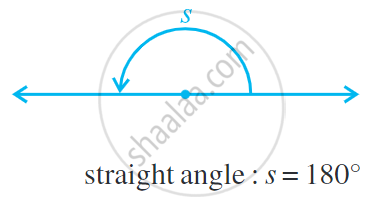5. An angle which is greater than 180° but less than 360° is called a reflex angle.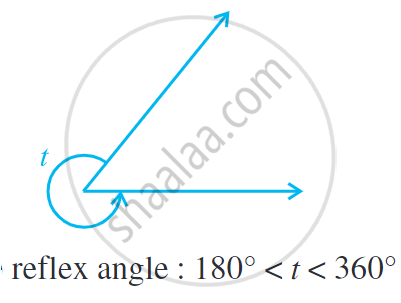6. The angle between the two sticks lie one upon the other is called a zero angle. The measure of the zero angles is written as 0°.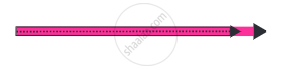7. Turning by two straight angles (or four right angles) in the same direction makes a full turn. This one complete turn is called one revolution. The angle for one revolution is a complete angle. Complete angle is one complete revolution or equal to 360°.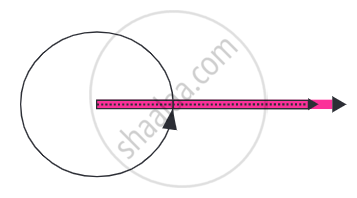If you would like to contribute notes or other learning material, please submit them using the button below.

### Shaalaa.com

Right Angle, Straight Angle, Complete Angle & Reflex Angle Part - 1 [00:16:11]
S
0%